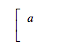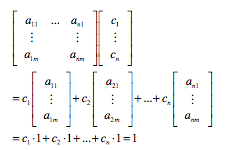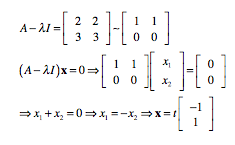## Saturday, April 28, 2012

### 5th Annual Math Circle Teacher Training Institute

Bob & Ellen Kaplan (founders of the Boston area math circles, and authors of Out of the Labyrinth: Setting Mathematics Free and many other intriguing math books),  along with Amanda Serenevy (founder of Riverbend Community Math Center), run a fabulous week-long Math Circle Teacher Training Institute, held on the beautiful campus of Notre Dame. This summer is their 5th annual Institute, and I'll be helping out for the first part of the week.

For only \$850, you get room and board... (The food is amazingly good for such a huge operation - not quite up to my personal organic, local, sustainable standards, but really yummy, with an incredible variety of choices.) ... and then you get to play with math all day. My friend, Ellen H, and I used to call it the math spa. (We went swimming every morning before breakfast.)

If you come, you'll also get to work with Tatiana Shubin and Anand Patel, who both have some serious math circle experience.

The first year I was amazed that I could do math all day long and never get tired of it. There's lots of freedom to explore in whatever way works best for you.

Schedule
Arrive: Sunday, July 8 (or as early as you can on Monday, July 9). Notre Dame is in South Bend, Indiana, 2 hours from Chicago.

Five days of math circle mania:

• we participate in math circles in the mornings,
• we run one math circle for kids late one afternoon, and watch our colleagues try it out on the other afternoons,
• we discuss the ins and outs of math circles and other fun ways of doing math in the early afternoons,
• we play with Amanda's collection of math toys and browse her collection of math books, whenever we want,
• we plot, plan, and socialize in between all that.
Depart: Saturday, July 14

Total cost: \$850

Next post: Rodi Steinig, a Math Circle Institute success story. She runs the math circle at the Talking Stick Learning Center, and blogs about it  here.

## Thursday, April 26, 2012

### Linear Algebra: Leading into the Eigen Stuff

[Note: If you read my blog for the posts on math for kids, I hope I haven't scared you off lately with all these posts about higher math. This is one of those; skip by me today, and I'll get back to the kids' stuff soon. Classes end in 3 weeks, and then I'll be working on my book, Playing With Math: Stories from Math Circles, Homeschoolers, and the Internet. Lots of goodies coming then.]

I wanted a good lead-in for this unit, and decided at the last minute to introduce Markov chains first. I wanted a fun application, but couldn't find anything simple online. (I was encouraged, though, to see how useful the concept seems to be.) I ended up using a problem from our text (#15 in 4.6). The problem gives a 'migration matrix' showing percent of Californians who stay in-state versus move away, and percent of people from other states who move to California versus not. Since we're in California, it seemed like a fun problem to look at.

I started (yesterday) with:
K said the numbers were decimals. I agreed, and added that they were pretty ugly, whereas we usually try to give ourselves nice simple numbers for our matrices. Pause... S said, "It adds up to 1." I wrote that under the first column, and they told me it was true of the second column and the vector too.

Then I told them it's called a probability vector when the entries are all positive or zero, and add up to 1. A matrix whose columns are all probability vectors is called a stochastic matrix or a transition matrix.

We did the multiplication and noticed that the result also added to 1. Hmm, will that always be true?
I wroteand asked them what the entry below a should be. They told me 1-a. I wrote a b in the next column, and they told me to put 1-b below it. I wrote the vector with a c, and they gave me the 1-c. We multiplied, getting:
Now we needed to add those 2 entries. Adding down, we saw ac+(1-a)c = c(1+1-a)=c*1=c, and b(1-c)+(1-b)(1-c) = (1-c)(b+1-b)=(1-c)*1. So all together we get c + (1-c) = 1. Sweet!

H asked if it worked for all sizes of matrices. I saw that he was being polite. He could have said, "Sue, your proof is only for a 2x2 matrix. Don't we want to prove it for all matrices?"  Whenever a student points out a mistake I've made, that's a donut point for the class. While recording the donut point, I said, "Oh, I see that I've only proved it for 2x2 matrices. What's your hunch, everyone, will it work for an nxn matrix?" Most of them thought it would work, and I asked them to try to prove the 3x3 case at home.  I had started to explain the next thing I wanted us to look at, when H said he thought he could prove it for nxn. I handed over the chalk and his proof was simple and elegant. I loved that it came from a student, instead of from me!

He started out with:
That would imply just the two columns. I told him how to change his subscripts, and he changed them. (We haven't used the double-subscripts much, if at all. We usually refer to the columns of a matrix as vectors, using [a1 a2 ...].) He now wrote:Isn't that beautiful?!

So we knew that any transition matrix times a probability vector will give a new probability vector. Next I told them what those numbers meant. I wrote "In 1989, 11.7% of the U.S. population lived in California."

They got that the vector was people in California versus out. (I was hoping someone might figure out what the matrix represented, too, but we didn't manage that.) I showed them what each entry in the matrix meant. The first column is about the population within California, and the second column is about the rest of the U.S. The top row is for people who end up in California, and the bottom row is for those who end up outside California. So over 98% of Californians keep their residence in the state, and under 2% move away each year. Multiplying our matrix P by the vector x0 gives a new value, x1, for the percent of the U.S. living in California in 1990. If the migration patterns remain the same, the percentages for 1991 will be given by P times x1 (or P2 times x0). We checked (on a student's smartphone) the current population percentage, and it was impressively close to the prediction given by  P23 times x0.

I finally introduced them to the phrase Markov chain. We create a chain of values by repeatedly multiplying by P. One of the questions raised by this process is whether you can find a vector, x, so that Px=x; this is called a steady state vector.

Today I proved that you could always find such a vector:
If  Px=x, then Px-x=0, or Px-Ix=0, or (P-I)x=0.
We need an x not equal to 0. Will it exist?
We can show that row reduction can produce a bottom row of P-I that is all zeros
Let a new version of the bottom row be all of the rows added together
(Each column added to 1, but has a 1 subtracted now, so adding all together = 0)
This allows for one free variable,
and implies the existence of a non-zero x satisfying the equation.
There is a related question we didn't address: Will other values of x (ever, sometimes, always) lead toward the steady state?

I moved on by generalizing our previous problem to:
For an nxn matrix A,
can we find a (non-zero) vector x and a scalar (number) c,
so that Ax=cx
Then I told them that our textbook, and everyone, used λ instead of c. No big deal, right? And yet, between the names used for parts of this topic (eigenvectors, eigenvalues, and eigenspaces) and the variable name used (a funky Greek letter, λ), I used to be pretty intimidated by this stuff.

We carried on valiantly, seeing that this equation could be played with much like the previous one:
Axx implies Axx=0, so Ax-λIx=0, giving us (A-λI)x=0
These won't be as easy to resolve as the P equations, since we have the added complication of the λ. But we have a tool for this. We know that we can only have non-zero solutions (x) if the matrix A-λI is not invertible. And we know that non-invertible matrices have determinant equal to 0. So we want
det(A-λI)=0. And that is called the characteristic equation.

I did one example to get us started:
det(A-λI)=0 gives us (5-λ)(6-λ)-6=0, which simplifies to λ2-11λ+24=0.
This factors to (λ-3)(λ-8)=0, so λ=3,8.

If λ=3, we getThe 3 and the 8 are called the eigenvalues. That last vector above is the eigenvector associated with 3, and you can talk about the eigenspace for which it forms a basis; there's an eigenvector for 8 (you find it, I'm tired), and its eigenspace. And we'll do more of this next week.

I hope my students are having as much fun as I am!